Saturday 31st July 2021
 CBSE Guess > Papers > Question Papers > Class XII > 2005 > Maths > Delhi Set-III MATHEMATICS 2005 (Set III—Delhi)
Except for the following questions, all the remaining questions have been asked in Set I and Set II SECTION - A  Q. 1. Find the probability of at most two tails or at least two heads in a toss of 3 coins. Q. 3. If A =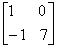, find K such that A2 -8A+K I =O. Q. 10. If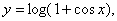prove that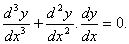Q. 14. Find the equation of the tangent to the curvewhich is parallel to the lineQ. 16. An open box with a square base is to be made out of a given iron sheet of area 27 sq. m. show that the maximum volume of the box is 13.5 cu. m. SECTION - B  Q. 21. A particle describes 650 m in seconds and its velocity at the end of 10 seconds is 80 m/sec. find the initial velocity and uniform acceleration. Q. 22. Forces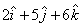act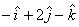on a particle. Determine the work done when the particle is displayed from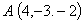to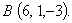Q. 25. Find the perpendicular distance of the point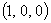frorm the line. SECTION- C  Q. 19. Solve the following linear programming problem graphically: Minimise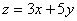subject to constraintsQ. 21. A man is known to speak the truth 3 out of 4 tomes. He throws a die and reports that it is a six. Find the probability that it is actually a six. Q. 23. Calculate the present value of an ordinary annuity of Rs. 10,000 per annum for 12 years, the rate of interest being 4% per annum compounded annually. [Use (1.04) -12 = 0.6252]. Maths 2005 Question Papers Class XII Delhi Outside Delhi Compartment Delhi Compartment Outside Delhi Set I Set I Set I Set I Set II Set II Set II Set II Set III Set III CBSE 2005 Question Papers Class XII